### Home > CC1 > Chapter 1 > Lesson 1.1.3 > Problem1-21

1-21.

Find the perimeter of each figure below. The markings on part (b) mean that the lines are parallel. The markings on part (e) show that all sides are the same length. As you find each perimeter, be sure to show your work.

1.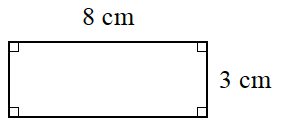• This shape is a rectangle. Find its perimeter. Remember that the perimeter is the total length of the border around the shape.

• Find the unknown lengths and label them on your diagram. Now try to find the perimeter without looking at the answer.

• $22 \text{ cm}$

• Be sure to show why the perimeter is $22$ cm.

1.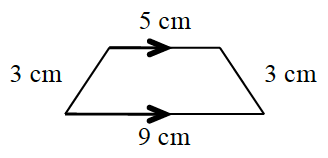• The perimeter is the total length of the border around the shape.

The perimeter is $3 \text{ cm} + 5 \text{ cm} + 3 \text{ cm} + 9 \text{ cm}$. Calculate this sum.

$20 \text{ cm}$. Can you show how you arrived at this answer?

1.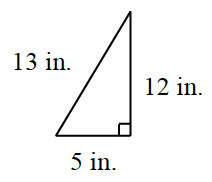See (a) and (b).

1.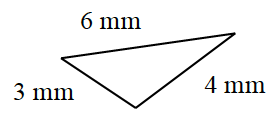See (a) and (b).

$13 \text{ mm}$

1.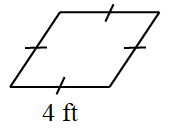The markings mean that all the sides are $4 \text{ ft}$ long.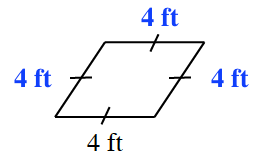1.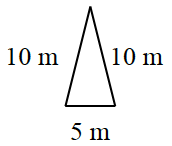• See (a) and (b).